Computing with the SIR Epidemic Model---Part 1

We will use the Euler method to solve the damped pendulum equation written as a systemfor initial conditions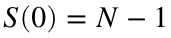,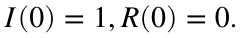I'm going to assume the disease is spreading on a small island with a population of 10000 people and that it starts when a single infected person arrives on the island..

Experiment 1: An outbreak

N = 10000;
S0=N-1;
I0=1;
beta=.0002;
gamma=1;

Set up time stepping

h = 1/100;
tfinal=20;
nsteps=tfinal/h;
t=linspace(0,tfinal,nsteps+1);

Initialize the variables

S=zeros(1,nsteps);
I = zeros(1,nsteps);
R = zeros(1,nsteps);
S(1)=S0; I(1)=I0;

Calculate the reproductive ratio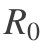We see that it is greater than one, so we expect an outbreak
R0=beta*N/gamma;
fprintf('The reproductive ratio is %0.2f.\n',R0)
The reproductive ratio is 2.00.

Loop through and run the Euler method

for k=1:nsteps
[Sprime,Iprime,Rprime]=SIRode(S(k),I(k),R(k),beta,gamma);
S(k+1) = S(k) + h * Sprime;
I(k+1) = I(k) + h * Iprime;
R(k+1) = R(k) + h * Rprime;
end
S1=S;I1=I; % Saving to plot at end

Plot the results as a funciton of time

plot(t,S,t,I,t,R);
xlabel('t'); legend('S','I','R')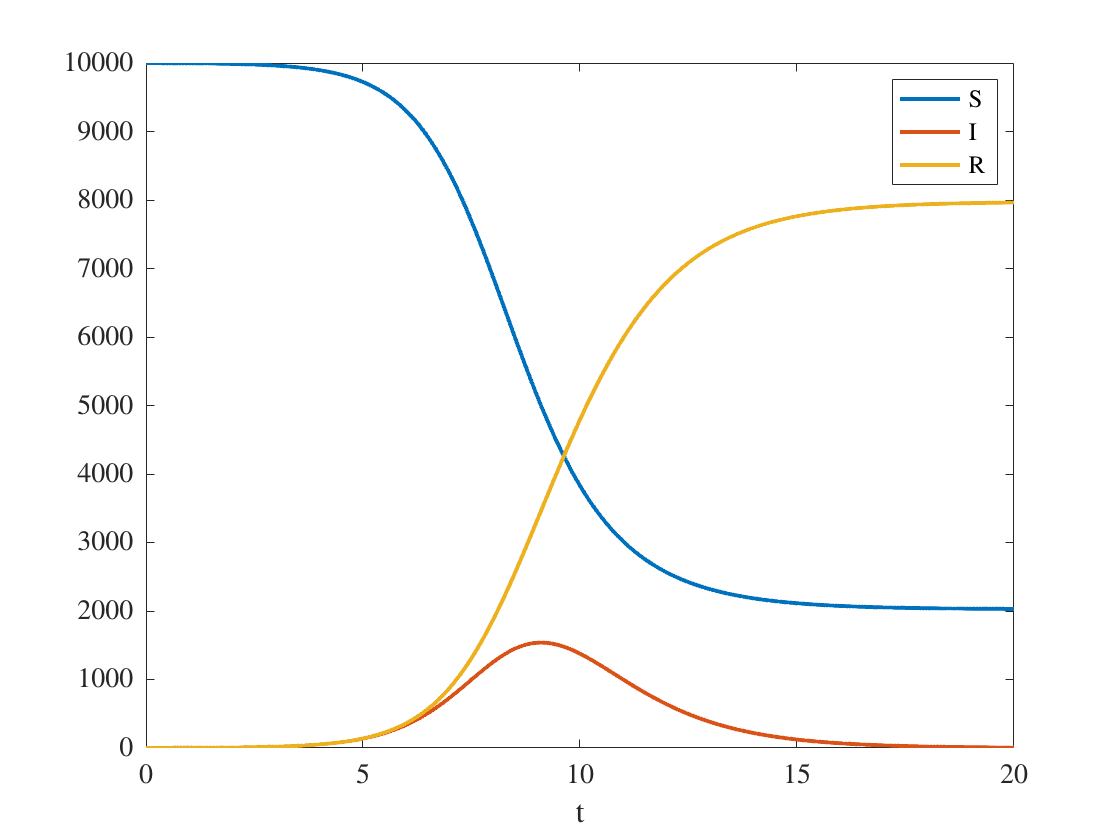Plot the solution in the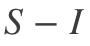phase plane

plot(S,I,S(1),I(1),'o')
xlabel('S');ylabel('I');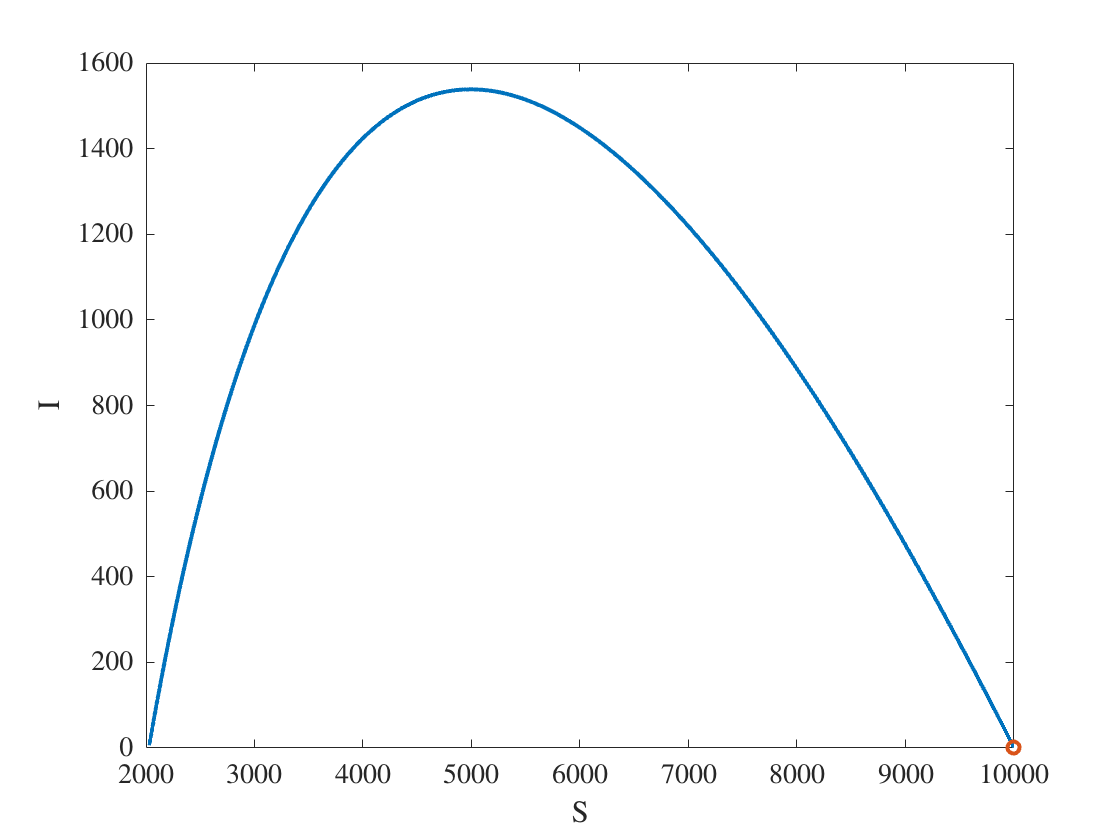fprintf('%0.1f%% of the population eventually caught the disease.\n',100*(R(end))/N)
79.6% of the population eventually caught the disease.
fprintf('At the epidemic''s peak, %0.1f%% of the population was infected.\n',100*max(I)/N)
At the epidemic's peak, 15.4% of the population was infected.

Experiment 2: A smaller outbreak

Now, suppose the government had implemented a stay-at-home order, which causes β to decrease to a avalue of 0.00125.

The epidemic now proceeds more slowly, so we have to run the simulation longer. We see that the total number of infected people, and the peak of the outbreak are significantly reduced.
beta=.000125;
tfinal=50;
nsteps=tfinal/h;
t=linspace(0,tfinal,nsteps+1);
S=zeros(size(t));
I = zeros(size(t));
S(1)=S0; I(1)=I0;
R0=beta*N/gamma;
fprintf('The reproductive ratio is %0.2f.\n',R0)
The reproductive ratio is 1.25.
for k=1:nsteps
[Sprime,Iprime,Rprime]=SIRode(S(k),I(k),R(k),beta,gamma);
S(k+1) = S(k) + h * Sprime;
I(k+1) = I(k) + h * Iprime;
R(k+1) = R(k) + h * Rprime;
end
S2=S;I2=I;
plot(t,S,t,I,t,R);
xlabel('t'); legend('S','I','R')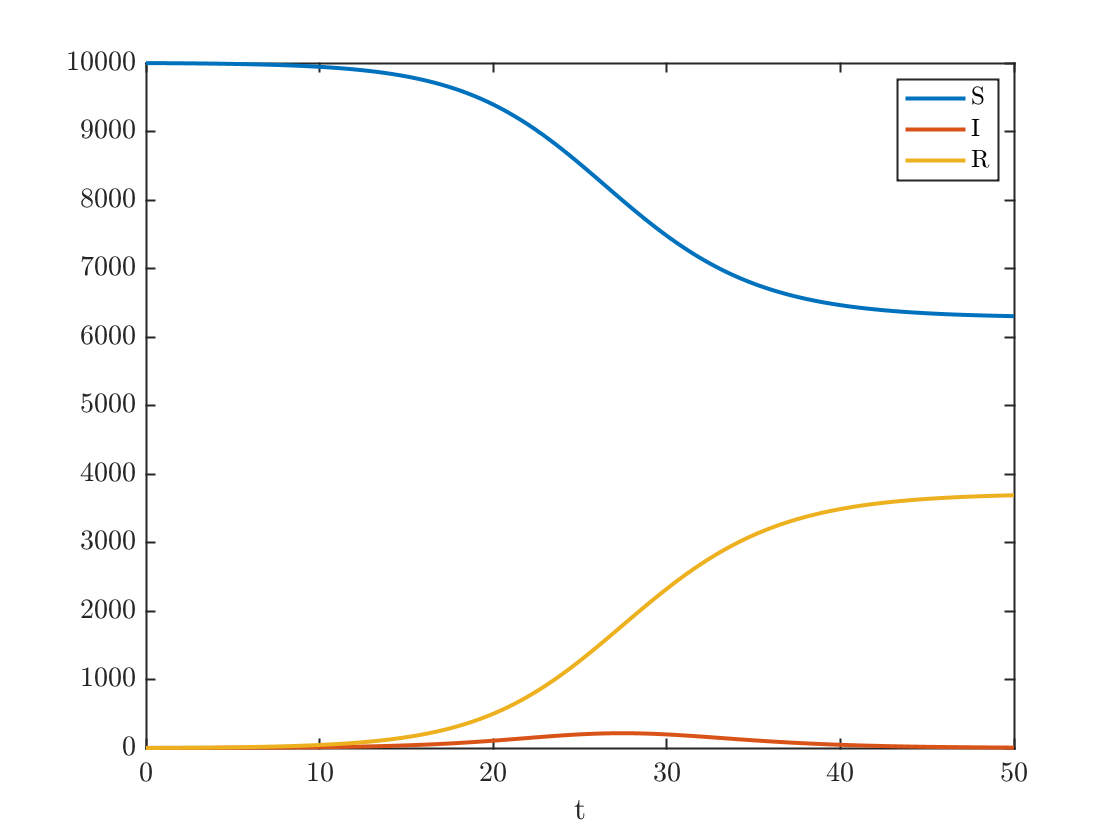Plot the solution in the phase plane

plot(S,I,S(1),I(1),'o')
xlabel('S');ylabel('I');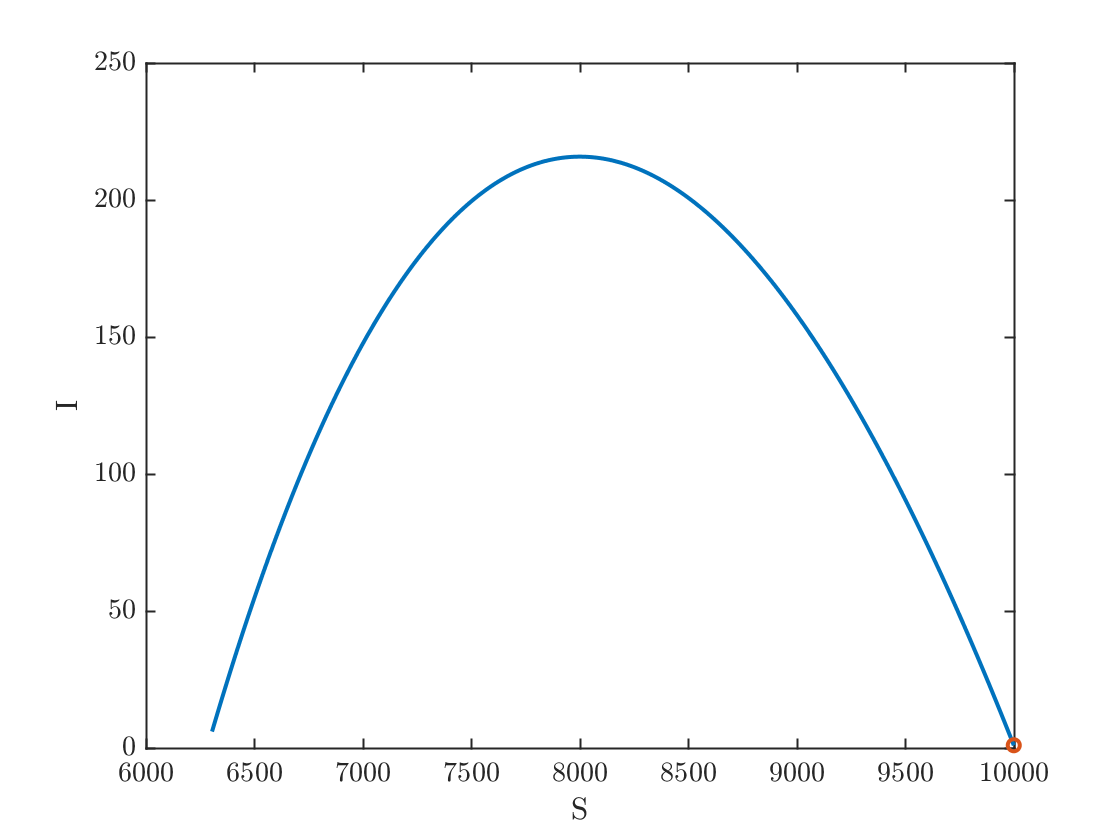fprintf('%0.1f%% of the population eventually caught the disease.\n',100*(R(end))/N)
36.9% of the population eventually caught the disease.
fprintf('At the epidemic''s peak, %0.1f%% of the population was infected.\n',100*max(I)/N)
At the epidemic's peak, 2.2% of the population was infected.

Experiment 3: A successful ingervention

Now, suppose the government had implemented a stay-at-home order, and people are disciplined about social-distancing and mask wearing, which causes β to decrease to a avalue of 0.0009.

In this case,and the pandemic never takes off. As a result, only 10 people ever get sick.
beta=.00009;
tfinal=50;
nsteps=tfinal/h;
t=linspace(0,tfinal,nsteps+1);
S = zeros(1,nsteps);
I = zeros(1,nsteps);
R = zeros(1,nsteps);
S(1)=S0; I(1)=I0; R(1)=0;
R0=beta*N/gamma;
fprintf('The reproductive ratio is %0.2f.\n',R0)
The reproductive ratio is 0.90.
for k=1:nsteps
[Sprime,Iprime,Rprime]=SIRode(S(k),I(k),R(k),beta,gamma);
S(k+1) = S(k) + h * Sprime;
I(k+1) = I(k) + h * Iprime;
R(k+1) = R(k) + h * Rprime;
end
plot(t,S,t,I,t,R);
xlabel('t'); legend('S','I','R')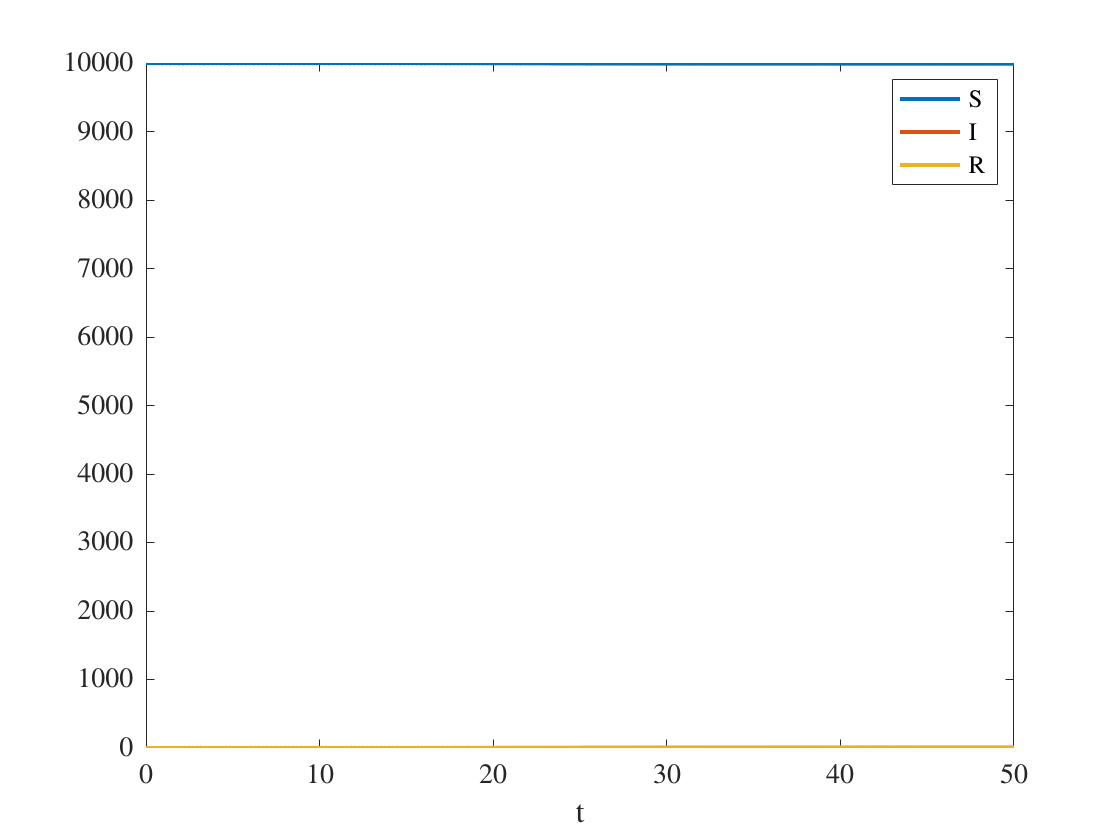Plot the solution in the phase plane

plot(S,I,S(1),I(1),'o')
xlabel('S');ylabel('I');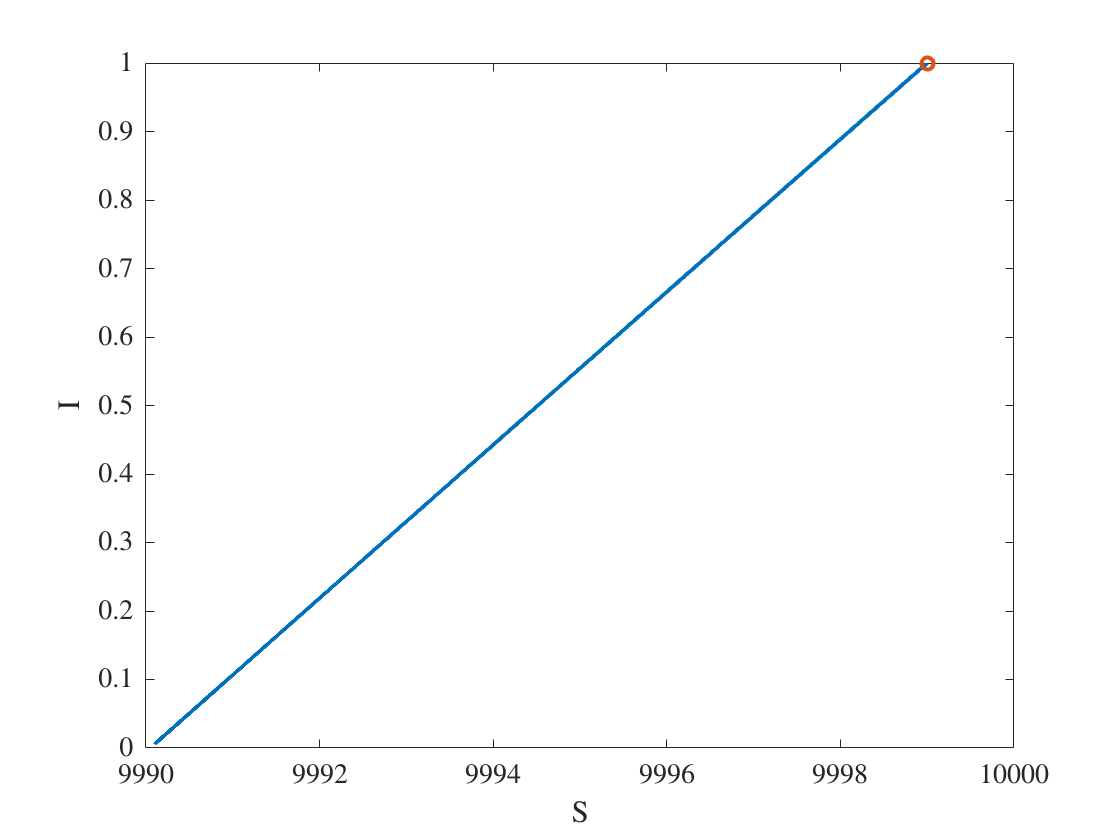fprintf('%0.1f%% of the population eventually caught the disease.\n',100*(R(end))/N)
0.1% of the population eventually caught the disease.
fprintf('At the epidemic''s peak, %0.1f%% of the population was infected.\n',100*max(I)/N)
At the epidemic's peak, 0.0% of the population was infected.
fprintf('In absolute terms, at the epidemic''s peak there were %i people infected and a total of %i people ever got infected.\n',ceil(max(I)),ceil(max(R)))
In absolute terms, at the epidemic's peak there were 1 people infected and a total of 10 people ever got infected.

Compare the three experiments

As computed above,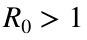in the first two experiments but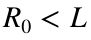in the third experiment, which correlates with our observation that outbreaks occur in the first two experiments but not in the third.
We plot the susceptible and infected populations in all three experiments. The result of experiment 3 barely visibile on this axis since the number of infected individuals goes to zero so fast and the the number of susceptible barely changes.
plot(S1,I1,S2,I2,S,I,'o')
xlabel('S');ylabel('I')
legend('Experiment 1','Experiment 2','Experiment 3 ')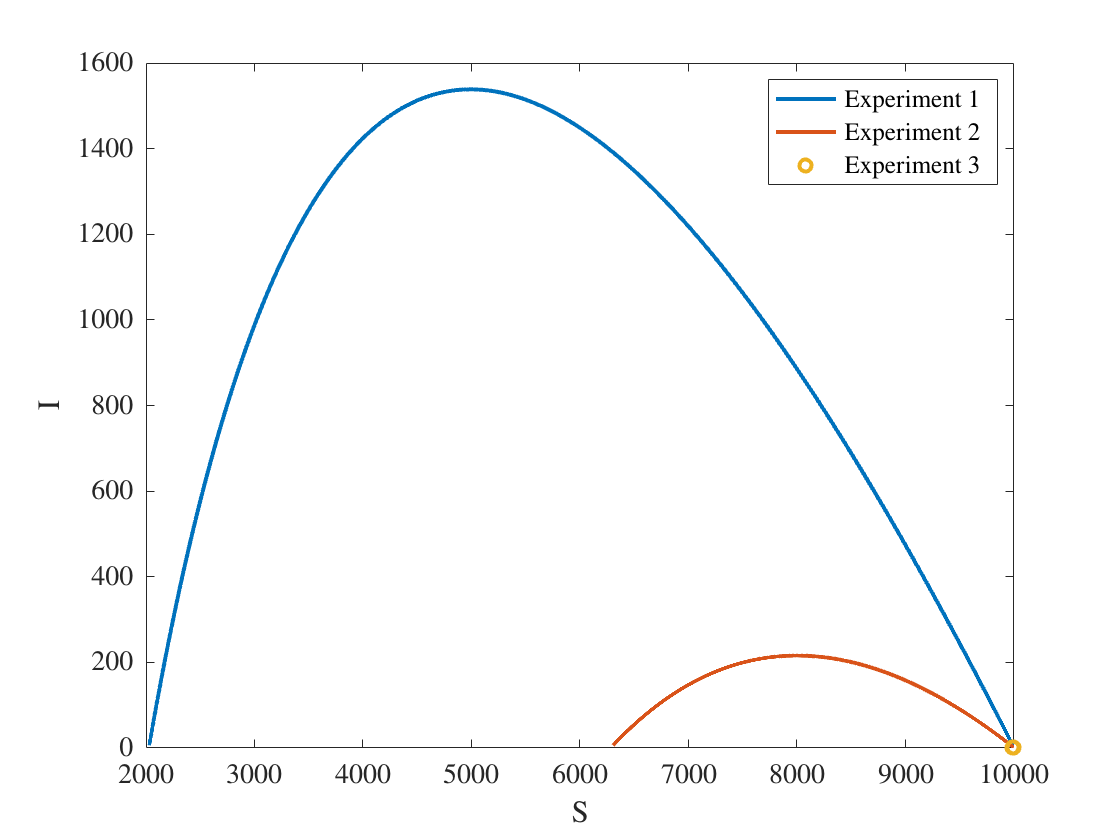Definition of the SIR ODE

function[Sprime,Iprime,Rprime]=SIRode(S,I,R,beta,gamma)
Sprime = -beta*S*I;
Iprime = beta*S*I - gamma*I;
Rprime = gamma*I;
end